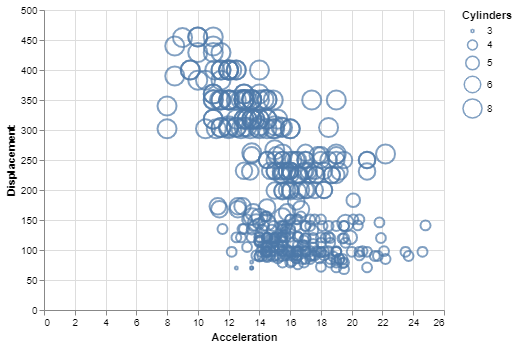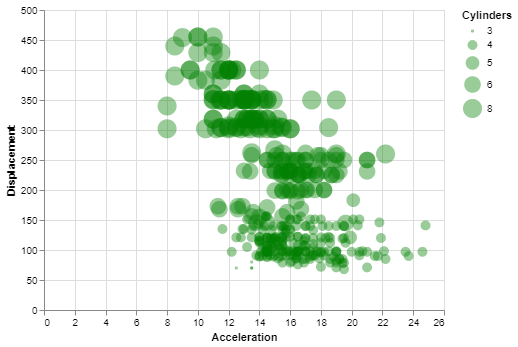Open in App
Not now

# How To Make Bubble plot with Altair in Python?

• Last Updated : 26 Nov, 2020

Prerequisite: Introduction to Altair in Python

Altair is a simple and easy to use statistical visualization library for python. It contains many types of built-in plots and various options to modify the properties and generate other plots. Bubble Plot is a very useful visualization for bivariate analysis of data with respect to a third variable. It is not readily available in the Altair library but can be made by doing some simple modifications to the scatter plot.

## What is a Bubble Plot?

Bubble Plot is basically a scatter plot between two variables/data columns where in place of the data points, there are bubbles/circles of varying sizes indicating the third variable. The third variable can be of a quantitative, ordinal, or nominal type, but the best type to be used in bubble plot is the ordinal type, i.e. data having a specific ordering. The legend shows which circle size corresponds to which data value.

A bubble plot can help us see the relationship between two variables with respect to a third variable. The bigger the bubble, the bigger value of data it corresponds to.

## Creating a Bubble Plot

To make a bubble plot, the user simply has to map a suitable variable from the dataset to the size encoding in a simple scatter plot.

The datasets used in these articles are from the Vega_datasets library.

## Python3

 `# Python3 program to illustrate ``# How to make a bubble plot``# using the altair library``   ` `# Importing altair and vega_datasets ``import` `altair as alt ``from` `vega_datasets ``import` `data ``   ` `# Selecting the cars dataset ``cars ``=` `data.cars() ``   ` `# Making the base scatter plot ``alt.Chart(cars).mark_point().encode( ``   ` `  ``# Map the sepalLength to x-axis ``    ``x ``=` `'Acceleration'``, ``   ` `  ``# Map the petalLength to y-axis ``    ``y ``=` `'Displacement'``,``   ` `  ``# Map the Cylinders variable to size``  ``# and specify it as a nominal variable``      ``size ``=` `'Cylinders:N'``)`

Output:Simple Bubble Plot using Altair

### Customizing the Bubble Plot

You can do the following customizations to the bubble plot:

• Color: You can change the default color of the bubbles by setting the color parameter of the mark_point() method.
• Opacity: You can change the default opacity of the bubbles by setting the opacity parameter of the mark_point() method. It ranges from 0 to 1.
• Filled: This is false by default, but you can change the filled parameter to true, thereby filling the bubble with the specified color.

Example:

## Python3

 `# Python3 program to illustrate``# how to customize a bubble plot`` ` `# Importing altair and vega_datasets``import` `altair as alt``from` `vega_datasets ``import` `data`` ` `# Selecting the cars dataset``cars ``=` `data.cars()`` ` `# Making the base scatter plot``# and adding the customizations``alt.Chart(cars).mark_point(color``=``'green'``,``                           ``filled``=``True``,``                           ``opacity``=``0.4``).encode(``   ` `    ``# Map the sepalLength to x-axis``    ``x``=``'Acceleration'``,``   ` `    ``# Map the petalLength to y-axis``    ``y``=``'Displacement'``,``   ` `    ``# Map the Cylinders variable to size``    ``# and specify it as a nominal variable``    ``size``=``'Cylinders:N'``)`

Output:Customized Bubble Plot using Altair

My Personal Notes arrow_drop_up